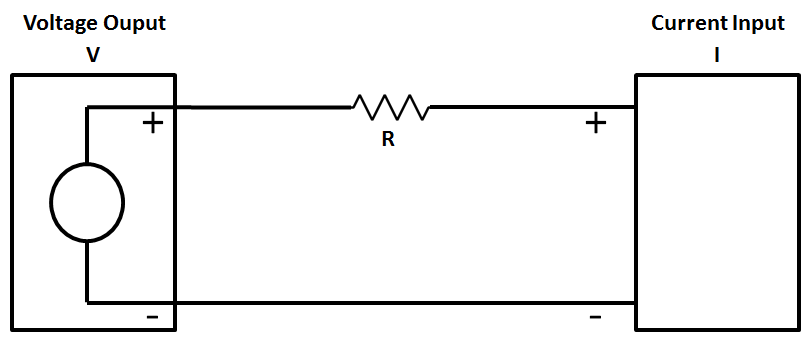Home » How to Convert Voltage to Current using Resistor ?
PLC Tutorials

How to Convert Voltage to Current using Resistor ?

In this article, we discuss about How to Convert Voltage to Current using Resistor with different examples like 0-10 VDC to 0-20 mA Conversion, 0-5 VDC to 0-20 mA Conversion.

Convert Voltage to Current

It is extremely simple to measure 0-10Vdc signal with a device that will measure only Current inputs. If Current input module available will accept a 0-20mA signal, but may not accept a 0-10Vdc signal directly.

Basically, Ohms law is used to calculate a resistor value in order to convert the 0-10Vdc signal to a current.Example : 0-10 VDC to 0-20 mA Conversion

Ohms law states: R = V/I where V is the Voltage, I is the current and R is the resistance

= 10V/0.020A = 500 OhmsI= V/R = 0/500   = 0mA
I= V/R = 10/500 = 0.02A = 20mA

Example : 0-5 VDC to 0-20 mA Conversion

Ohms law states: R = V/I where V is the Voltage, I is the current and R is the resistance

= 5V/0.020A = 250 OhmsI= V/R = 0/250   = 0mA

I= V/R = 5/250 = 0.02A = 20mA

Note:-

• To avoid damage you must ensure that the external current source has short-circuit protection in all conductor cases.
• The external resistor is a source of error because of its dependency on temperature and its inaccuracy.
• In order to obtain measuring results that are as precise as possible it is recommended to use resistors with tolerances that are as small as possible.

Credits : myplctechnology blog

1 commentSeptember 17, 2019 at 2:46 am

You didn’t consider the internal resistance of the input.

This website uses cookies to improve your experience. We'll assume you're ok with this, but you can opt-out if you wish. Accept Read More

WordPress Image Lightbox

Send this to a friend